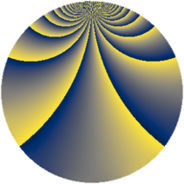# Properties

 Label 1089.1.kLevel $1089$ Weight $1$ Character orbit 1089.k Rep. character $\chi_{1089}(118,\cdot)$ Character field $\Q(\zeta_{10})$ Dimension $8$ Newform subspaces $1$ Sturm bound $132$ Trace bound $0$

# Related objects

## Defining parameters

 Level: $$N$$ $$=$$ $$1089 = 3^{2} \cdot 11^{2}$$ Weight: $$k$$ $$=$$ $$1$$ Character orbit: $$[\chi]$$ $$=$$ 1089.k (of order $$10$$ and degree $$4$$) Character conductor: $$\operatorname{cond}(\chi)$$ $$=$$ $$11$$ Character field: $$\Q(\zeta_{10})$$ Newform subspaces: $$1$$ Sturm bound: $$132$$ Trace bound: $$0$$

## Dimensions

The following table gives the dimensions of various subspaces of $$M_{1}(1089, [\chi])$$.

Total New Old
Modular forms 104 24 80
Cusp forms 8 8 0
Eisenstein series 96 16 80

The following table gives the dimensions of subspaces with specified projective image type.

$$D_n$$ $$A_4$$ $$S_4$$ $$A_5$$
Dimension 8 0 0 0

## Trace form

 $$8 q - 2 q^{4} + O(q^{10})$$ $$8 q - 2 q^{4} - 2 q^{16} + 2 q^{25} + 2 q^{49} - 2 q^{64} - 4 q^{91} + 4 q^{97} + O(q^{100})$$

## Decomposition of $$S_{1}^{\mathrm{new}}(1089, [\chi])$$ into newform subspaces

Label Dim $A$ Field Image CM RM Traces $q$-expansion
$a_{2}$ $a_{3}$ $a_{5}$ $a_{7}$
1089.1.k.a $8$ $0.543$ 8.0.64000000.1 $D_{4}$ $$\Q(\sqrt{-3})$$ None $$0$$ $$0$$ $$0$$ $$0$$ $$q-\beta _{2}q^{4}-\beta _{7}q^{7}-\beta _{1}q^{13}+\beta _{4}q^{16}+\cdots$$